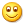• fakefish
• 5681 访问

从 React 说起

<ListItem item-id="123" />

ListItem({itemId: 123})

什么是纯函数

• 其结果只能从它的参数的值来计算
• 不能依赖能被外部操作改变的数据
• 不能改变外部状态

var a = 1;
increment = funciton() {
return a + 1;
}

increment = function(a) {
return a + 1;
}

其他

[1,2,3,4,5].map(function(num) {
if(num % 2 != 0) return;

num *= 3;
num = 'num is ' + num;

return num;
})

[1,2,3,4,5].filter(n => n % 2 == 0)
.map(n => n * 3)
.map(n => 'num is ' + n)

time = 5
positions = [1,1,1]

do (time) ->
time -= 1
postions.each (pos, i) ->
if Math.random() > 0.7
positions[i] += 1

console.log '-' + pos

time = 5
positions = [1,1,1]

move = ->
for pos, i in positions
if Math.random() > 0.3
positions[i] += 1

drawCar = (pos) ->
console.log '-' + pos

run = ->
time -= 1
move()

draw = ->
for pos in positions
drawCar pos

do (time) ->
run()
draw()

move = (positions) -> positions.map (x) -> Math.random() * 0.3 ? x + 1 : x

drawCar = (pos) -> '-' + pos

run = (state) -> { time: state.time  - 1, positions: move( state.positions )}

draw = (state) -> state.positions.map( drawCar )

race = (state) ->
draw(state)
if state.time then race(move(state))

race({time: 5, positions: [1,1,1]})

函数响应式编程

\$input.on('input', function() {
if(stop()) {
if(length > 2) {
if(value != lastValue) {
search();
}
}
}
});

Observable
.from(\$input, 'input')
.map(function(e) {
return e.target.value;
})
.filter(function(text) {
return text.length > 2;
})
.debounce(750)
.distinctUntilChanged()
.flatMapLatest(searchPromise)
.subscribe(function(data) {
show(data);
})

var dragTarget = document.getElementById('dragTarget');

// Get the three major events
var mouseup   = Rx.Observable.fromEvent(dragTarget, 'mouseup');
var mousemove = Rx.Observable.fromEvent(document,   'mousemove');
var mousedown = Rx.Observable.fromEvent(dragTarget, 'mousedown');

var mousedrag = mousedown.flatMap(function (md) {

// calculate offsets when mouse down
var startX = md.offsetX, startY = md.offsetY;

// Calculate delta with mousemove until mouseup
return mousemove.map(function (mm) {
mm.preventDefault();

return {
left: mm.clientX - startX,
top: mm.clientY - startY
};
}).takeUntil(mouseup);
});

// Update position
var subscription = mousedrag.subscribe(function (pos) {
dragTarget.style.top = pos.top + 'px';
dragTarget.style.left = pos.left + 'px';
});

ReactiveX is more than an API, it's an idea and a breakthrough in programming. It has inspired several other APIs, frameworks, and even programming languages.

抽奖

Lottery
.filter(()=> {
return true;
})
.wait(()=> {
console.log('wait for click');
return true;
})
.until(()=> {
return new Promise((resolve, reject) => {
setTimeout(()=>{
console.log('user clicked');
resolve(true);
}, 2000)
});
})
.filter((e) => {
console.log('event: ' + e);
return true;
})
.lottery(() => {
return new Promise((resolve, reject) => {
console.log('client request');
setTimeout(()=>{
console.log('server respones');
Math.random().toFixed(1) > 0.5 ? resolve({data: 'hello'}): reject('net work error');
}, 2000)
});
})
.done((d) => {
console.log('lottery result: ' + d.data);
return d;
})
.error((e) => {
console.error('error: '+e);
})
.filter((d) => {
console.log('last filter: ' + d);
return true;
})
.end()

/**
* 抽奖的事件流抽象描述
* 需要把所有的操作变成promise对象
*/
let Lottery = (() => {
let Lottery = {};
let actions = [];
let errorAction;
let actionNames = ['filter', 'wait', 'until', 'lottery', 'done', 'error'];
for(name of actionNames) {
if(name == 'error') {
Lottery[name] = function(action) {
errorAction = action;
return this;
}
} else {
Lottery[name] = function(action) {
actions.push(action);
return this;
}
}
}

Lottery.end = () => {
doActions(actions);
};

let doActions = async (actions) => {
let ret = null;
try {
for(let action of actions) {

ret = await new Promise((resolve, reject) => {
let result = action(ret);
if(typeof result == void 0 || result == false) reject();
resolve(result);
});
}
} catch(e) {
errorAction(e);
}
return ret;
};

return Lottery;
})();共收到1条回复

•如果我猜错的话，您应该是好搜的王佳裕工程师，对吗？回复此楼